# 10-327/Homework Assignment 1

Problem. Let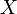$X$ and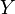$Y$ be topological spaces and let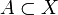$A\subset X$ and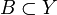$B\subset Y$ be subsets thereof. Using only the definitions in terms of continuity of certain functions, show that the topology induced on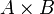$A\times B$ as a subset of the product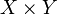$X\times Y$ is equal to the topology induced on it as a product of subsets of$X$ and of$Y$. You are allowed to use the fact that two topologies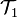${\mathcal T}_1$ and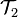${\mathcal T}_2$ on some set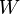$W$ are equal if and only if the identity map regarded as a map from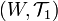$(W, {\mathcal T}_1)$ to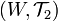$(W, {\mathcal T}_2)$ is a homeomorphism. Words like "open sets" and "basis for a topology" are not allowed in your proof.
Note on Question 8, Page 92. (Added 8:00AM, September 27). One should think that "describe" for verbal things is like "simplify" for formula-things. The topologies in question were given by a verbal description; the content of the question is that you should be giving a simpler one, and the best is if it is of the form "the topology in question is the trivial topology", or something like that. Note that the resulting topology may also depend on the direction of the line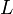$L$, so you may wish to divide your answer into parts depending on that direction.